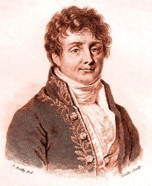# Fourier Series

Jean Baptiste Joseph Fourier,a French mathematician and a physicist; was born in Auxerre, France. He initialized Fourier series, Fourier transforms and their applications to problems of heat transfer and vibrations. The Fourier series, Fourier transforms and Fourier's Law are named in his honour.Jean Baptiste Joseph Fourier (21 March 1768 – 16 May 1830)

## Fourier series

To represent any periodic signal x(t), Fourier developed an expression called Fourier series. This is in terms of an infinite sum of sines and cosines or exponentials. Fourier series uses orthoganality condition.

### Fourier Series Representation of Continuous Time Periodic Signals

A signal is said to be periodic if it satisfies the condition x (t) = x (t + T) or x (n) = x (n + N).

Where T = fundamental time period,

ω0= fundamental frequency = 2π/T

There are two basic periodic signals:

$x(t) = \cos\omega_0t$ (sinusoidal) &

$x(t) = e^{j\omega_0 t}$ (complex exponential)

These two signals are periodic with period $T= 2\pi/\omega_0$.

A set of harmonically related complex exponentials can be represented as {$\phi_k (t)$}

$${ \phi_k (t)} = \{ e^{jk\omega_0t}\} = \{ e^{jk({2\pi \over T})t}\} \text{where} \,k = 0 \pm 1, \pm 2 ..n \,\,\,.....(1)$$

All these signals are periodic with period T

According to orthogonal signal space approximation of a function x (t) with n, mutually orthogonal functions is given by

$$x(t) = \sum_{k = - \infty}^{\infty} a_k e^{jk\omega_0t} ..... (2)$$

$$= \sum_{k = - \infty}^{\infty} a_kk e^{jk\omega_0t}$$

Where $a_k$= Fourier coefficient = coefficient of approximation.

This signal x(t) is also periodic with period T.

Equation 2 represents Fourier series representation of periodic signal x(t).

The term k = 0 is constant.

The term $k = \pm1$ having fundamental frequency $\omega_0$, is called as 1st harmonics.

The term $k = \pm2$ having fundamental frequency $2\omega_0$, is called as 2nd harmonics, and so on...

The term $k = ±n$ having fundamental frequency $n\omega0$, is called as nth harmonics.

### Deriving Fourier Coefficient

We know that $x(t) = \Sigma_{k=- \infty}^{\infty} a_k e^{jk \omega_0 t} ...... (1)$

Multiply $e^{-jn\omega_0 t}$ on both sides. Then

$$x(t)e^{-jn\omega_0 t} = \sum_{k=- \infty}^{\infty} a_k e^{jk \omega_0 t} . e^{-jn\omega_0 t}$$

Consider integral on both sides.

$$\int_{0}^{T} x(t) e^{jk \omega_0 t} dt = \int_{0}^{T} \sum_{k=-\infty}^{\infty} a_k e^{jk \omega_0 t} . e^{-jn\omega_0 t}dt$$

$$\quad \quad \quad \quad \,\, = \int_{0}^{T} \sum_{k=-\infty}^{\infty} a_k e^{j(k-n) \omega_0 t} . dt$$

$$\int_{0}^{T} x(t) e^{jk \omega_0 t} dt = \sum_{k=-\infty}^{\infty} a_k \int_{0}^{T} e^{j(k-n) \omega_0 t} dt. \,\, ..... (2)$$

by Euler's formula,

$$\int_{0}^{T} e^{j(k-n) \omega_0 t} dt. = \int_{0}^{T} \cos(k-n)\omega_0 dt + j \int_{0}^{T} \sin(k-n)\omega_0t\,dt$$

$$\int_{0}^{T} e^{j(k-n) \omega_0 t} dt. = \left\{ \begin{array}{l l} T & \quad k = n \\ 0 & \quad k \neq n \end{array} \right.$$

Hence in equation 2, the integral is zero for all values of k except at k = n. Put k = n in equation 2.

$$\Rightarrow \int_{0}^{T} x(t) e^{-jn\omega_0 t} dt = a_n T$$

$$\Rightarrow a_n = {1 \over T} \int_{0}^{T} e^{-jn\omega_0 t} dt$$

Replace n by k.

$$\Rightarrow a_k = {1 \over T} \int_{0}^{T} e^{-jk\omega_0 t} dt$$

$$\therefore x(t) = \sum_{k=-\infty}^{\infty} a_k e^{j(k-n) \omega_0 t}$$

$$\text{where} a_k = {1 \over T} \int_{0}^{T} e^{-jk\omega_0 t} dt$$